SSAT Elementary Level Math : How to find the decimal equivalent of a fraction

Example Questions

Example Question #61 : Numbers And Operations

What isequal to in decimal form?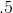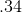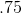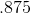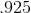Explanation:

To find the decimal equivalent of a fraction, simply divide the top number (numerator) by the bottom number (denominator). In this problem, 3 divided by 4 equals .75!

Example Question #62 : Numbers And Operations

What isequal to in decimal form?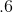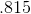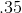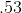Explanation:

To find the decimal equivalent of a fraction, simply divide the top number (numerator) by the bottom number (denominator). In this problem, 3 divided by 5 equals .6!

Example Question #63 : Numbers And Operations

What is the decimal equivalent of?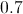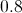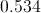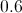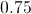Explanation:

Multiply the top and bottom of the fraction by a number that would make the denominator 100. In our case, this is 25.

The product of 4 and 25 is 100.

The product of 3 and 25 is 75.

Since 100 has 2 zeroes, write down 75 with the decimal point two spaces to the right: 0.75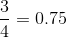Example Question #64 : Numbers And Operations

What is 0.21 as a fraction?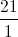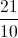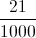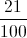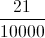Explanation:

The number of digits after the decimal point in 0.21 is two. This means the denominator will be 1 followed by two zeroes: 100

Place the two digits after the decimal point above the 100, as the numerator: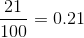Example Question #65 : Numbers And Operations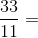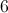Explanation:

Recall your multiplication tables: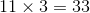Since multiplication and division are inverse operations, it follows that: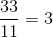Example Question #71 : Numbers And Operations

What is the fractionequal to in decimal form?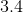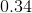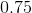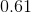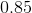Explanation:

To find the decimal equivalent of a fraction, divide the top number (numerator) by the bottom number (denominator).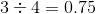Example Question #72 : Numbers And Operations

What is the fraction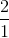equal to in decimal form?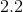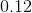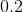Explanation:

To find the decimal equivalent of a fraction, divide the top number (numerator) by the bottom number (denominator).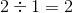Example Question #73 : Numbers And Operations

What is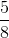equal to in decimal form?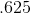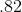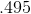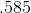Explanation:

To find the decimal equivalent of a fraction, divide the top number (numerator) by the bottom number (denominator). In this problem,divided byequals!

Example Question #74 : Numbers And Operations

What is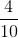equal to in decimal form?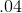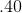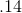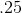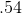Explanation:

To find the decimal equivalent of a fraction, divide the top number (numerator) by the bottom number (denominator). In this problem,divided byequals!

Example Question #75 : Numbers And Operations

What isequal to in decimal form?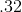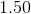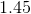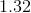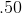To find the decimal equivalent of a fraction, divide the top number (numerator) by the bottom number (denominator). In this problem,divided byequals!